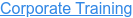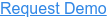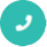#### 15+ Categories. 500+ IT Courses to Learn.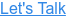# Data Science in Python Interview Questions and Answers

by sonia, on May 18, 2017 6:28:25 PMAre you trying for a Python job? Here are the top frequently asked interview questions and answers to step-on the python interview. Dive into these Python interview questions and answers and see just how well-versed you are in this Python language.

## Q4. Which library would you prefer for plotting in Python language: Seaborn or Matplotlib?

Ans: Matplotlib is the python library used for plotting but it needs lot of fine-tuning to ensure that the plots look shiny. Seaborn helps data scientists create statistically and aesthetically appealing meaningful plots. The answer to this question varies based on the requirements for plotting data.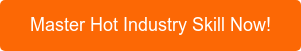## Q9. Which method in pandas.tools.plotting is used to create scatter plot matrix?

Ans 5,6,7,8,9: Scatter_matrix.

## Q10. How can you check if a data set or time series is Random?

Ans: To check whether a dataset is random or not use the lag plot. If the lag plot for the given dataset does not show any structure then it is random.

## Q14. Which is the standard data missing marker used in Pandas?

Ans: NaN## Q18. Which Python library would you prefer to use for Data Munging?

Ans: 15,16,17,18: Pandas.

## Q19. Write the code to sort an array in NumPy by the nth column?

Ans: Using argsort () function this can be achieved. If there is an array X and you would like to sort the nth column then code for this will be x[x [: n-1].argsort ()]

## Q21. Which python library is built on top of matplotlib and Pandas to ease data plotting?

Ans: 20,21: Seaborn

Ans: Bootstrap

## Q25. What is pylab?

Ans: 23,24,25: A package that combines NumPy, SciPy and Matplotlib into a single namespace.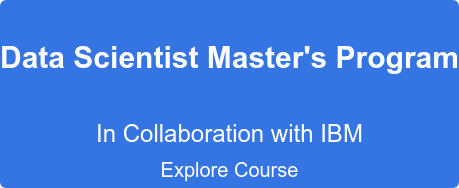## Q26. Which python library is used for Machine Learning?

Ans: SciKit-Learn

Learn Data Science in Python to become an Enterprise Data Scientist

## Q27. How can you copy objects in Python?

Ans: The functions used to copy objects in Python are:

• Copy.copy () for shallow copy
• Copy.deepcopy () for deep copy
However, it is not possible to copy all objects in Python using these functions.  For instance, dictionaries have a separate copy method whereas sequences in Python have to be copied by ‘Slicing’.

## Q28. What is the difference between tuples and lists in Python?

Ans: Tuples can be used as keys for dictionaries i.e. they can be hashed. Lists are mutable whereas tuples are immutable - they cannot be changed. Tuples should be used when the order of elements in a sequence matters. For example, set of actions that need to be executed in sequence, geographic locations or list of points on a specific route.

## Q29.What is PEP8?

Ans: PEP8 consists of coding guidelines for Python language so that programmers can write readable code making it easy to use for any other person, later on.

## Q30. Is all the memory freed when Python exits?

Ans: No it is not, because the objects that are referenced from global namespaces of Python modules are not always de-allocated when Python exits.

## Q31. What does _init_.py do?

Ans: _init_.py is an empty py file used for importing a module in a directory. _init_.py provides an easy way to organize the files. If there is a module maindir/subdir/module.py,_init_.py is placed in all the directories so that the module can be imported using the following command-
import  maindir.subdir.module

## Q32. What is the different between range () and xrange () functions in Python?

Ans: range () returns a list whereas xrange () returns an object that acts like an iterator for generating numbers on demand.

## Q33. How can you randomize the items of a list in place in Python?

Ans: Shuffle (lst) can be used for randomizing the items of a list in Python.

## Q34. What is a pass in Python?

Ans: Pass in Python signifies a no operation statement indicating that nothing is to be done.

## Q35. If you are gives the first and last names of employees, which data type in Python will you use to store them?

Ans: You can use a list that has first name and last name included in an element or use Dictionary.

## Q36. What happens when you execute the statement mango=banana in Python?

Ans: A name error will occur when this statement is executed in Python.

## Q38. Optimize the below python code:

word = 'word'
print word.__len__ () ?

Ans: print ‘word’._len_ ()

## Q39. What is monkey patching in Python?

Ans: Monkey patching is a technique that helps the programmer to modify or extend other code at runtime. Monkey patching comes handy in testing but it is not a good practice to use it in production environment as debugging the code could become difficult.

## Q40. Which tool in Python will you use to find bugs if any?

Ans: Pylint and Pychecker. Pylint verifies that a module satisfies all the coding standards or not. Pychecker is a static analysis tool that helps find out bugs in the course code.

## Q41. How are arguments passed in Python- by reference or by value?

Ans: The answer to this question is neither of these because passing semantics in Python are completely different. In all cases, Python passes arguments by value where all values are references to objects.

## Q42. You are given a list of N numbers. Create a single list comprehension in Python to create a new list that contains only those values which have even numbers from elements of the list at even indices. For instance if list has an even value the it has be included in the new output list because it has an even index but if list has an even value it should not be included in the list because it is not at an even index.

[x for x in list [1::2] if x%2 == 0]

Ans: The above code will take all the numbers present at even indices and then discard the odd numbers.

## Q43. Explain the usage of decorators.

Ans: Decorators in Python are used to modify or inject code in functions or classes. Using decorators, you can wrap a class or function method call so that a piece of code can be executed before or after the execution of the original code. Decorators can be used to check for permissions, modify or track the arguments passed to a method, logging the calls to a specific method, etc.

## Q44. How can you check whether a pandas data frame is empty or not?

Ans: The attribute df.empty is used to check whether a data frame is empty or not.

## Q45. What will be the output of the below Python code:

def multipliers ():
return [lambda x: i * x for i in range (4)]
print [m (2) for m in multipliers ()]

Ans: The output for the above code will be [6, 6,6,6]. The reason for this is that because of late binding the value of the variable i is looked up when any of the functions returned by multipliers are called.

## Q46. What do you mean by list comprehension?

Ans: The process of creating a list while performing some operation on the data so that it can be accessed using an iterator is referred to as List Comprehension.
Example:
[ord (j) for j in string.ascii_uppercase]
[65, 66, 67, 68, 69, 70, 71, 72, 73, 74, 75, 76, 77, 78, 79, 80, 81, 82, 83, 84, 85, 86, 87, 88, 89, 90]

## Q47. What will be the output of the below code:

word = ‘aeioubcdfg'
print word [:3] + word [3:]?

Ans: The output for the above code will be: ‘aeioubcdfg'.
In string slicing when the indices of both the slices collide and a “+” operator is applied on the string it concatenates them.

## Q48. list= [‘a’,’e’,’i’,’o’,’u’]print list [8:]

Ans: The output for the above code will be an empty list []. Most of the people might confuse the answer with an index error because the code is attempting to access a member in the list whose index exceeds the total number of members in the list. The reason being the code is trying to access the slice of a list at a starting index which is greater than the number of members in the list.

## Q49. What will be the output of the below code:

Ans: def foo (i= []):
i.append (1)
return i
>>> foo ()
>>> foo ()

The output for the above code will be:

[1, 1]
Argument to the function foo is evaluated only once when the function is defined. However, since it is a list, on every all the list is modified by appending a 1 to it.

## Q50. Can the lambda forms in Python contain statements?

Ans: No, as their syntax is restrcited to single expressions and they are used for creating function objects which are returned at runtime.
This list of questions for Python interview questions and answers is not an exhaustive one and will continue to be a work in progress. Let us know in comments below if we missed out on any important question that needs to be up here.